# Flow Rate Calculator

## Online Mass and Volumetric Flow Rate Calculator suitable for liquids like Water or Gases like AirHe is a Process Control Lover experienced in complex and high profitable automation systems in the chemical and plastics industry. For a few years now, he has been an Associate Professor at the University' Fluid Mechanics Department and Director of a Postgraduate Degree in Industrial Control.

## How to calculate volumetric fluid flow rate?

Flow is measured as a volume or mass per unit time. This calculator let you calculate the flow rate of any liquid or gas fluid starting from different linear, volumetric flow rate, mass flow rate or normal flow rate.

Using this calculator you can solve questions such as:

How to convert mass flow rate to volumetric flow rate?

Where can I find a easy velocity to mass flow rate converter?

How to convert volumetric flow rate to mass flow rate?

What is the difference between flow and pressure?

Flow measurement is one of the most important operations inside a chemical or process plant. Through the flow measurement we can know the quantity of raw material consumed, intermediate products, energies used (steam, water, fuels) and processed products. Flow is defined as the mass or volume of fluid flowing through a pipe or conduit per unit of time.

Optimum performance of some equipment and operations requiere specific flow rates. The cost of many liquids and gases are based on the measurement flow through a pipeline making it necessary to accurately measure and control the rate of flow for accounting purposes.

Proper installation of any flowmeter in fluid systems is essential in relation to piping layout, streamline-flow conditions, and accesibility to the device and its associated instruments and connections

### How does this volumetric flow rate calculator work?

You simply fill in the cells with a white background and press the RESULTS button.

The cells Tagname, Plant, Area and Notes are not necessary to perform the calculation but they are necessary if you want to export the obtained calculations to a results spreadsheet.

The following video will show how this free volumetric flow rate calculator works:

The State cell allows you to select the state of matter. Depending on the state of the matter, the necessary cells are enabled or disabled to perform the calculation. When you select Liquid, the calculation let you specify the density of the liquid to be able to calculate the mass flow rate of the fluid. If you want to use this calculator as an air flow rate calculator, you have to select the Gas option and then define the Pressure, Temperature of the working conditions and the Molecular Weight of the gas. When you select the Gas option the density is calculated by the calculator depeding on Pressure, Temperature and MW.

For example, if you want to calculate the compressed air flow rate you have to introduce a MW of 28.97. Other MW for typical gases can he found here.

Tagname
Plant
Area

## Fluid & Pipe Data

Fluid
State
Density [Kg/m3] (rho)
Operating Temperature [ºC] (T)
Operating Pressure [bar a] (P)
Molecular Weight (MW)
Pipe Diameter [mm] (D)
Starting data:
Velocity in Pipe [m/s]

Pipe Area [m2] (A)
Velocity in Pipe [m/s] (vp)
Volumetric Flow [m3/h] (qv)
Volumetric Flow [CFM] (qvc)
Mass Flow [Kg/s] (qm)
Mass Flow [Kg/h] (qmh)
Normal Flow [Nm3/h] (qn)
Standard Flow [SCFM] (qs)
Normal Conditions 20 °C and 101.325 kPa, Standard Conditions 14.696 psi absolute and 68 ºF

We don't like spam either, but if we want to keep you informed we need you to provide us a minimal contact information. Thanks for your collaboration.

## Information and Definitions

Tagname

Tagname of the instrument. This is the identifier of the field device, which is normally given to the location and function of the instrument.

If you want to discover more details about Functional Naming visit our dedicated page to this subject, P&ID Diagram Basics – Part 3 - Functional Identification and Naming Conventions.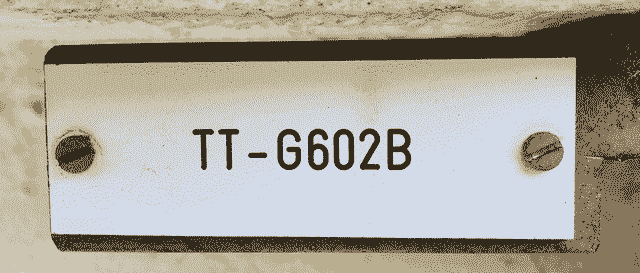Plant, Area and Notes

Information Referred to the physical installation of the instrument. Plant and Process Area where the instrument is installed. Usually, in order to easily locate the different phases of the production process, the entire chemical plant is usually divided into different areas. The areas may have names related to the phase of the production process, such as Pyrolysis, or they may be related to the type of service they produce, such as Compressed Air.

Notes about the instrument. You can use the Notes field to add adtional information related to your calculation, like for example GARDEN HOSE or SOAKER HOSE.

These fields are not required by the calculator, but in case you decide to download your results this is information useful in order to organize your information.

Fluid

Fluid Name or Composition. Fluid is called a type of continuous medium formed by some substance whose molecules have only a weak force of attraction.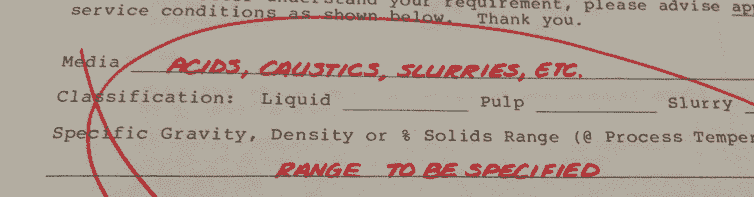A fluid is a set of particles that are held together by weak cohesive forces and the walls of a container; The term encompasses liquids and gases. This information is not relevant for the calculation but you can use this cell to define the fluid flowing through the pipe, like for example compressed air flow.

State

State of the matter. It could be Liquid or Gas.

In case you select Liquid, you need the define the density of the fluid. This information is required for the calculation to calculate the different flow rates. In case you need to use this calculator like a water meter flow rate calculator, you can keep 1000 in the density cell. We have created a dedicated page named Density of Common Liquids that contains a table of several densities with it's own reference temperature. The page can he found here.

Temperature (T)

Operating Temperature of the fluid in Celsius units. Temperature has two effects on volume. A higher temperature means a less dense gas and higher flows, but when this higher flow is corrected to base temperature, the base flow is less. This cell is only enabled when you select Gas as the State of the matter. In case you don't know which is the operating temperature you can leave it to 20 Celsius degrees.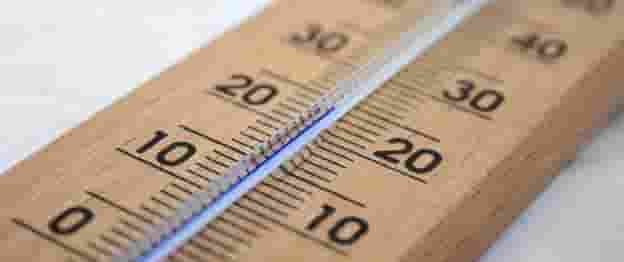Operating Temperature of the fluid in Celsius units.

Pressure (P)

Operating Pressure of the flui in Bar units. Pressure has two effects on volume. The higher pressure makes the gas denser so less volume flows through the meter. However, when the volume is expanded to base pressure, the volume is increased.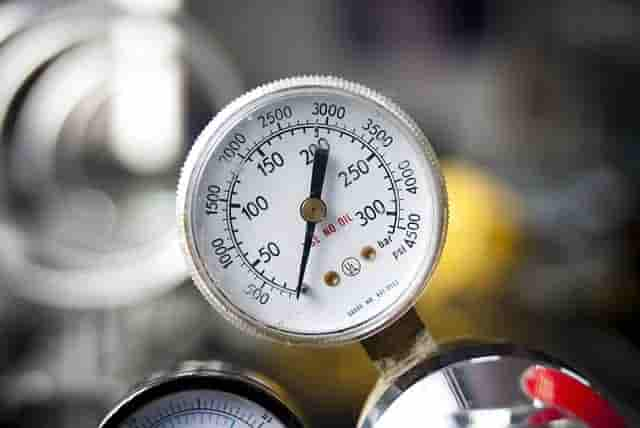Avogadro was the one who determined that under standard conditions the volume that a mole of any gaseous substance occupies is always the same. This value is 22.4 liters. The volume of a mole of any gas is known as the molar volume. For example: 1 mole of hydrogen, 1 mole of nitrogen or water vapor, chlorine, carbon dioxide, etc. they will always occupy 22.4 liters in standard conditions. If these conditions change (they are no longer 1 atmosphere or 273 K), the volume will also change.

Density (rho)

Density is the relation of mass and volume.The density of a material varies with temperature and pressure. This variation is typically small for solids and liquids but much greater for gases.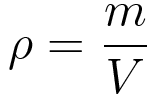...where rho is density, m is mass and V is volume.

Molecular Weight (MW)

Mass of a molecule of any pure substance, the value of which is the sum of the atoms that make it up.

Pipe Diameter (D)

Inside diameter of the pipe. All process calculations are based on the volume of the pipe which is the function of internal diameter of the pipe. As per standards, any pipe is specified by two non-dimensional numbers Nominal Diameter (in Inches as per American Standards or mm as per European standards) and Schedule (40, 80, 160,...). The outer diameter of the pipe is the diameter of outer surface of the pipe.

Velocity in Pipe or Velocity Flow Rate (vp)

Velocity is a measure of speed and direction of an object. When related to fluids it is the rate of flow of fluid particles in a pipe. The average rate flow is used in flow calculations. The units of flow are normally feet per second (fps), feet per minute )fpm), meters per second (mps), and so on. This calculator can be used to measure the steam pipe velocity calculator....where V is Velocity in Pipe or Velocity Flow Rate, Q is Volumetric Flow Rate and Area is Pipe Surface.

Volumetric Flow Rate (qv)

Volumetric flow is often deduced by knowing the cross sectional area of the fluid. Most volumetric flow equipment measures the velocity and calculates the volumetric flow based on a constant cross seciotnal area. Volumetric flow is usually represented with Q letter. The volumetric flow rate units are usually m3/h or m3/s. Below you will find the volumetric flow formula:...where Q is Volumetric Flow Rate, rho is Density and W is Mass Flow Rate.

Volumetric Flow Conversion : mass flow Rate can be converted to volumetric flow rate using the above formula. Now, the difference between volumetric flow and mass flow rate can be easily understood observing the equation that relates both flows.

Mass Flow Rate (qm)

Mass of a substance which passes per unit of time. Mass flow in Kg/s units, flowing through the pipe. Mass flow is usually represented with W letter. Below you will find the mass flow formula: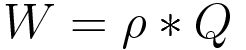...where W is Mass Flow Rate, rho is Density and Q is Volumetric Flow Rate.

Normal Flow Rate (qm)

Standard or normal conditions are used as reference values ​​in thermodynamics of gases. To specify the gas volume, Normal or Standard temperature and pressure conditions are generally used. The reason is very simple, the volume of a constant number of moles of gas depends on the measurements of temperature and pressure.

## Flow Rate Calculator References

1. International Organization of Standards (ISO 5167-1). 2003. Measurement of fluid flow by means of pressure differential devices inserted in circular cross-section conduits running full — Part 1: General principles and requirements.

2. International Organization of Standards (ISO 5167-2) 2003. Measurement of fluid flow by means of pressure differential devices inserted in circular cross-section conduits running full -- Part 2: Orifice plates.

3. American Society of Mechanical Engineers (ASME). 2001. Measurement of fluid flow using small bore precision orifice meters. ASME MFC-14M-2001.

4. U.S. Dept. of the Interior, Bureau of Reclamation, 2001 revised, 1997 third edition, Water Measurement Manual.

5. Michael Reader-Harris (2015) Orifice Plates and Venturi Tubes.

6. Miller, R. W., Flow Measurement Handbook, 3rd ed., McGraw-Hill, New York, 1996.

7. American Gas Association, AGA Gas Measurement Manual, American Gas Association, New York.

8. Wikipedia

9. Corrosionpedia

10. Orifice Plates and Venturi Tubes (2015) - Michael Reader-Harris

11. EMERSON Fundamentals of Orifice Meter Measurement

12. Search Data Center

## Any question regarding the Liquid or Gas Flow Rate Calculator?

### If you have any question regarding the Liquid or Gas Flow Rate Calculator calculator, please don't hesitate to get in touch ... Anyway you can have a look at our frequently asked questions page, there you will find a solution to the most common questions.#### What is the Difference Between Absolute, Gauge and Differential Pressure?

by Iñaki Eseberri, 2016/09/21

Any good instrumentation technician needs to know the difference between the basic types of pressure measurement.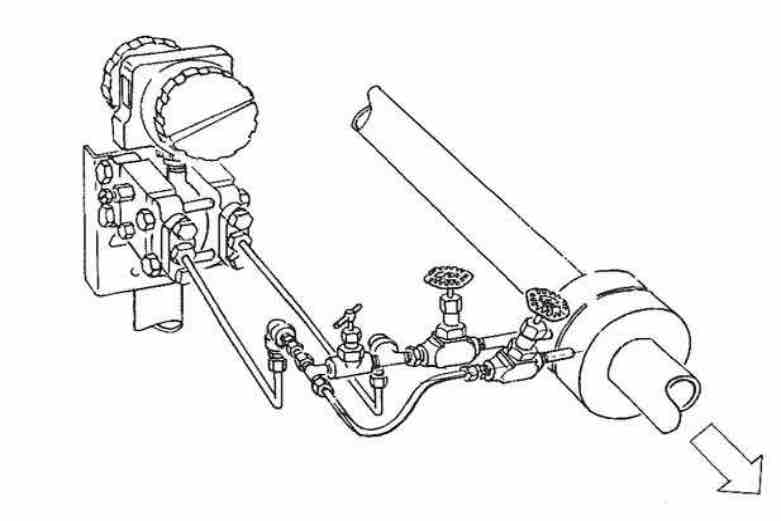#### Orifice Plate Installation Guidelines

by Iñaki Eseberri, 2018/03/09

This post is mainly a set of rules for the orifice plate installation like an orifice flow meter installation guidelines. In order that a flow instrument may accurately read flow, it’s necessary that the installation comply with certain rules.#### Difference between Actual, Standard and Normal Flows

by Iñaki Eseberri, 2018/03/29

Gas density changes with pressure and temperature, so the use of standardized volumes when referring to quantities of gas is mandatory. Standardized volumes are commonly used in different types of industries which uses gas as raw material. The volum of gas ...

x